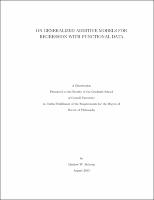## On Generalized Additive Models For Regression With Functional Data##### Abstract
The focus of this dissertation is the introduction of the functional generalized additive model (FGAM), a novel regression model for association studies between a scalar response and a functional predictor. The FGAM extends the commonly used functional linear model (FLM), offering greater flexibility while still being simple to interpret and easy to estimate. The link-transformed mean response is modelled as the integral with respect to t of F {X (t), t} where F ([MIDDLE DOT], [MIDDLE DOT]) is an unknown, bivariate regression function and X (t) is a functional covariate. Compare this with the FLM which has F {X (t), t} = [beta] (t)X (t), where [beta] (t) is an unknown coefficient function. Rather than having an additive model in some projection of the data, the model incorporates the functional predictor directly and thus can be viewed as the natural functional extension of generalized additive models. The first part of the dissertation shows how to estimate F ([MIDDLE DOT], [MIDDLE DOT]) using tensorproduct B-splines with roughness penalties. Fast, stable methods are used to fit the FGAM and I discuss how approximate confidence bands can be constructed for the true regression surface. Additional functional predictors can be included with little added difficulty. The performance of the estimation procedure and the confidence bands is evaluated using simulated data and I compare FGAM's predictive performance with other competing scalar-on-function regression alternatives, including the popular functional linear model. I illustrate the usefulness of the approach through an application to brain tractography, where X (t) is a signal from diffusion tensor imaging at position, t, along a tract in the brain. In one example, the response is disease-status (case or control) and in a second example, it is the score on a cognitive test. R code for performing estimation, plotting, and prediction for the FGAM is explained and is available in the package refund on CRAN. Frequently in practise, only incomplete, noisy versions of the functions one wishes to analyze are observed. The estimation procedure used in the first part of the thesis requires that the functional predictors be noiselessly observed on a regular grid. In the second part of the dissertation, I restrict attention to the identity link-Gaussian error case and develop a Bayesian version of FGAM. This approach allows for the functional covariates to be sparsely observed and measured with error. I consider both Monte Carlo and variational Bayes methods for jointly fitting the FGAM with sparsely observed covariates and recovering the true functional predictors. Due to the complicated form of the model posterior distribution and full conditional distributions, standard Monte Carlo and variational Bayes algorithms cannot be used. As such, the work should be of independent interest to applied Bayesian statisticians. The numerical studies demonstrate the benefits of the proposed algorithms over a two-step approach of first recovering the complete trajectories using standard techniques and then fitting a functional regression model. In a real data analysis, the methods are applied to forecasting closing price for items being auctioned on the online auction website eBay. Finally, in the third part of the thesis I propose and compare several different procedures for testing when a scalar on function regression relationship is truly nonlinear. By using an alternative parametrization for the FGAM as a mixed model, it is shown how the functional linear model can be represented as a simple mixed model nested within the FGAM. Using this representation, I then consider two types of tests, those based on restricted likelihood ratio tests for zero variance components in mixed models and those involving Bayes factors where we use generalizations of g-priors as priors for the random effects coefficients. The methods are general and can also be applied to testing for interactions in a multivariate additive model or for testing for no effect in the functional linear model. The performance of the proposed tests is assessed on simulated data and in an application to measuring diesel truck emissions, where strong evidence of nonlinearities in the relationship between the functional predictor and the response are found.
2013-08-19
##### Keywords
Functional data analysis; Generalized additive models; Scalar on function regression
Ruppert, David
##### Committee Member
Matteson, David
Hooker, Giles J.
Resnick, Sidney Ira
##### Degree Discipline
Operations Research
##### Degree Name
Ph. D., Operations Research
##### Degree Level
Doctor of Philosophy
##### Types
dissertation or thesis